# Separate First and Last Names – Excel & Google Sheets

This tutorial will demonstrate how to separate first and last names in Excel and Google Sheets.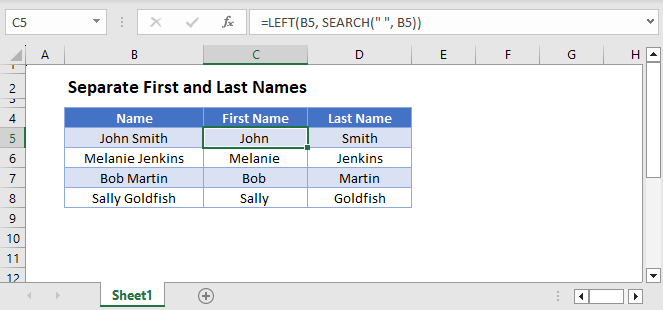## Separate First Name

First, to separate the first name from the name, we can use the LEFT and SEARCH functions.

``=LEFT(B3, SEARCH(" ", B3))``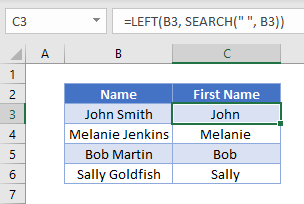## Separate Last Name

We then use the SEARCH and MID functions to get the last name from the name.

``=MID(B3, SEARCH(" ", B3)+1, 999)``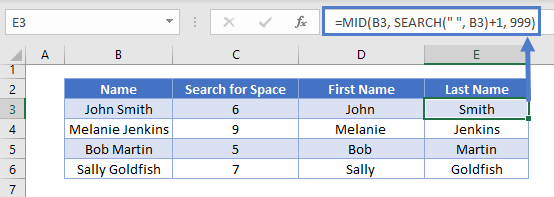We will walkthrough this below.

### SEARCH Function (First Name)

First, we used the SEARCH Function to find the position of the space between the first and last names.

``=SEARCH(" ", B3)``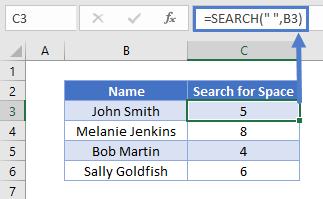### LEFT Function (First Name)

Next, we use the LEFT Function to return the characters before the space (the first name):

``=LEFT(B3,C3)``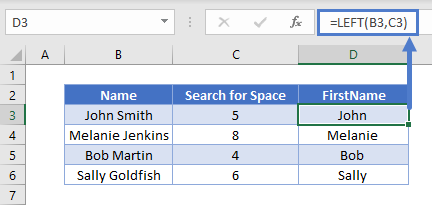Combining these functions yields the original formula for the first name:

``=LEFT(B3, SEARCH(" ", B3))``Next we will walkthrough how to extract the last name.

In the next section, we will use the SEARCH and MID functions to extract characters from the middle of a text string.

### SEARCH Function (Last Name)

First, we used the SEARCH Function to find the position of the space between the first and last names.

``=SEARCH(" ", B3)``We then add one onto the value returned by this formula to get the starting position of the last name.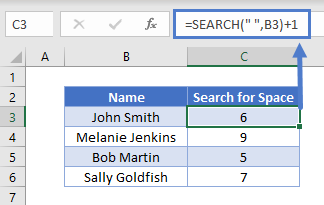### MID Function (Last Name)

Next we use the MID Function to return all the characters after the space plus 1 (the last name)

``=MID(B3, C3+1, 999)``

Combining these 2 functions gives us the original formula for the last name.

``=MID(B3, SEARCH(B3, " ")+1, 999)``### Easy Excel Automation## Middle Names

Next, we will discuss how to separate names that include middle names or initials.

The calculation for the first name remains unchanged.

To extract a middle name or initial from a name, we would need to use the RIGHT, SEARCH and LEN functions to get the last name from the name string.  We are also going to incorporate the TRIM function to trim any spaces on either side of the text string.

``````=RIGHT(B4,LEN(B4)-SEARCH(" ",B4)-LEN(TRIM(MID(B4,SEARCH(" ",B4,1)
+1,SEARCH(" ",B4,SEARCH(" ",B4,1)+1)-SEARCH(" ",B4,1))))-1)``````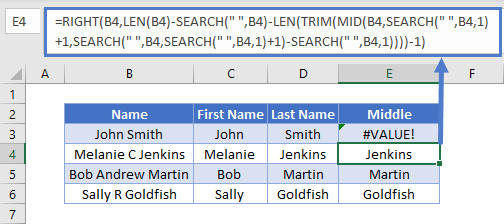This formula will only work if there is more than one space in the text string.  If there is only one space, an error with #VALUE would be returned.

To solve this problem, for names without middle names or initials, we can use the original formula using the MID and SEARCH Functions.

We could then combine the 2 formulas using the IFERROR Function.

``````=IFERROR(RIGHT(B3,LEN(B3)-SEARCH(" ",B3)-LEN(TRIM(MID(B3,SEARCH(" ",B3,1)+1,
SEARCH(" ",B3,SEARCH(" ",B3,1)+1)-SEARCH(" ",B3,1))))-1),MID(B3,SEARCH(" ",B3)+1,999))``````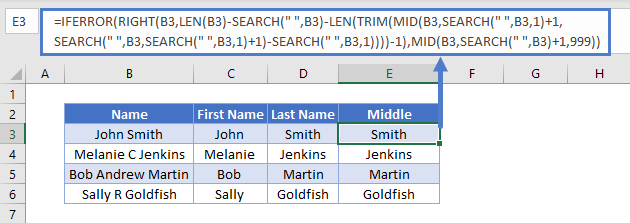We could then use the MID and LEN functions to obtain the middle name or initial.

``=MID(B3,LEN(C3)+1,LEN(B3)-LEN(C3&D3))``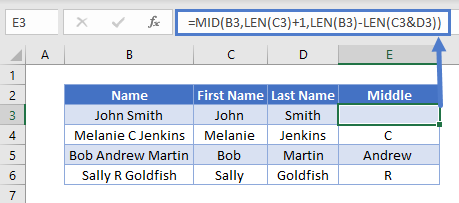## Separate First and Last Names in Google Sheets

All the examples explained above work the same in Google sheets as they do in Excel.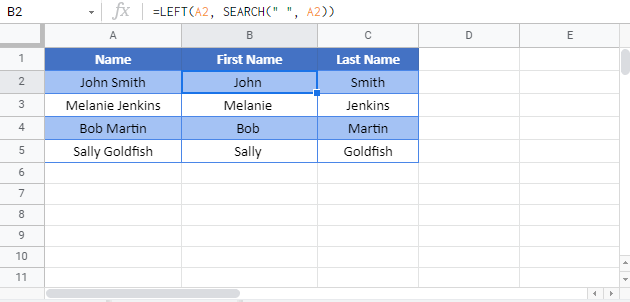### Excel Practice Worksheet

Practice Excel functions and formulas with our 100% free practice worksheets!